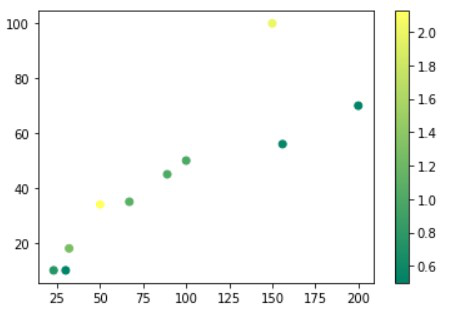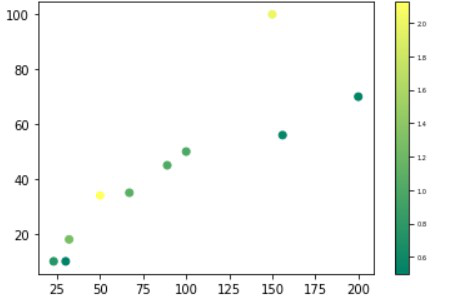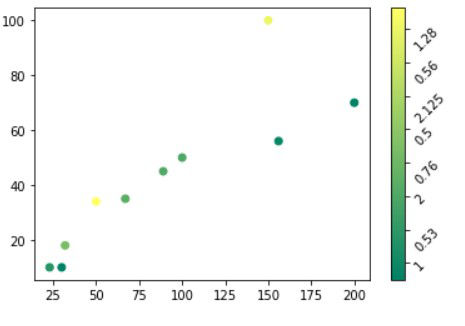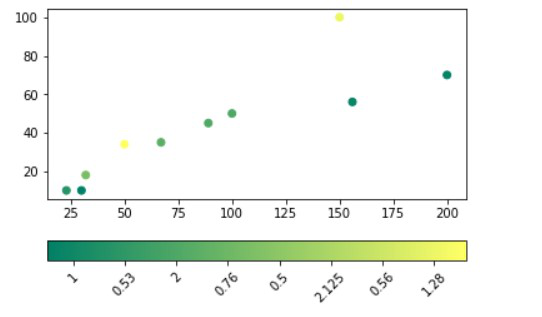GeeksforGeeks App
Open AppBrowser
Continue

# How to change colorbar labels in matplotlib ?

In this article, we are going to see how to change color bar labels in matplotlib using Python.

The colorbar() function is used to plot the color bar which belongs to the pyplot module of matplotlib adds a colorbar to a plot indicating the color scale.

Syntax: matplotlib.pyplot.colorbar(mappable=None, cax=None, ax=None, **kwarg)

Parameters:

• ax: This parameter is an optional parameter and it contains Axes or list of Axes.
• **kwarg(keyword arguments): This parameter is an optional parameter and are of two kinds:
• colorbar properties:
• extend:{‘neither’, ‘both’, ‘min’, ‘max’} makes pointed end(s) for out-of-range values.
• label:The label on the colorbar’s long axis.
• ticks:None or list of ticks or Locator.

Returns:colorbar which is an instance of the class ‘matplotlib.colorbar.Colorbar’.

## Create a simple colorbar for demonstration

To create the colorbar we will use color() methods, for this, we will create the dataset and then use a scatterplot for demonstration.

## Python3

 `# Python Program illustrating``# pyplot.colorbar() method``import` `numpy as np``import` `matplotlib.pyplot as plt` `# Dataset``# List of total number of items purchased``# from each products``purchaseCount ``=` `[``100``, ``200``, ``150``, ``23``, ``30``, ``50``,``                ``156``, ``32``, ``67``, ``89``]` `# List of total likes of 10 products``likes ``=` `[``50``, ``70``, ``100``, ``10``, ``10``, ``34``, ``56``, ``18``, ``35``, ``45``]` `# List of Like/Dislike ratio of 10 products``ratio ``=` `[``1``, ``0.53``, ``2``, ``0.76``, ``0.5``, ``2.125``, ``0.56``,``        ``1.28``, ``1.09``, ``1.02``]` `# scatterplot``plt.scatter(x``=``purchaseCount, y``=``likes, c``=``ratio, cmap``=``"summer"``)` `plt.colorbar()``plt.show()`

Output:## Method 1: Change labels font size in a color label

To change the label’s font size we will use ax.tick_params() methods which increase the font of the labels.

## Python3

 `# Python Program illustrating``# pyplot.colorbar() method``import` `numpy as np``import` `matplotlib.pyplot as plt` `# Dataset``# List of total number of items purchased``# from each products``purchaseCount ``=` `[``100``, ``200``, ``150``, ``23``, ``30``, ``50``,``                ``156``, ``32``, ``67``, ``89``]` `# List of total likes of 10 products``likes ``=` `[``50``, ``70``, ``100``, ``10``, ``10``, ``34``, ``56``, ``18``, ``35``, ``45``]` `# List of Like/Dislike ratio of 10 products``ratio ``=` `[``1``, ``0.53``, ``2``, ``0.76``, ``0.5``, ``2.125``, ``0.56``,``        ``1.28``, ``1.09``, ``1.02``]` `# scatterplot``plt.scatter(x``=``purchaseCount, y``=``likes, c``=``ratio,``            ``cmap``=``"summer"``)` `plt.colorbar().ax.tick_params(labelsize``=``10``)` `plt.show()`

Output:## Method 2: Change and Rotate the position of the label

To rotate the colorbar labels we will use set_xticklabels() and set_yticklabels() methods for horizontal and vertical.

Example 1: Changing position horizontally

## Python3

 `# Python Program illustrating``# pyplot.colorbar() method``import` `numpy as np``import` `matplotlib.pyplot as plt` `# Dataset``# List of total number of items purchased``# from each products``purchaseCount ``=` `[``100``, ``200``, ``150``, ``23``, ``30``, ``50``,``                ``156``, ``32``, ``67``, ``89``]` `# List of total likes of 10 products``likes ``=` `[``50``, ``70``, ``100``, ``10``, ``10``, ``34``, ``56``, ``18``, ``35``, ``45``]` `# List of Like/Dislike ratio of 10 products``ratio ``=` `[``1``, ``0.53``, ``2``, ``0.76``, ``0.5``, ``2.125``, ``0.56``,``        ``1.28``, ``1.09``, ``1.02``]` `# scatterplot``plt.scatter(x``=``purchaseCount, y``=``likes, c``=``ratio,``            ``cmap``=``"summer"``)` `plt.colorbar().ax.set_ylabel(``'Label'``,``                             ``rotation``=``120``)` `plt.show()`

Output:Example 2: Changing position vertically

## Python3

 `# Python Program illustrating``# pyplot.colorbar() method``import` `numpy as np``import` `matplotlib.pyplot as plt` `# Dataset``# List of total number of items purchased``# from each products``purchaseCount ``=` `[``100``, ``200``, ``150``, ``23``, ``30``, ``50``,``                ``156``, ``32``, ``67``, ``89``]` `# List of total likes of 10 products``likes ``=` `[``50``, ``70``, ``100``, ``10``, ``10``, ``34``, ``56``, ``18``, ``35``, ``45``]` `# List of Like/Dislike ratio of 10 products``ratio ``=` `[``1``, ``0.53``, ``2``, ``0.76``, ``0.5``, ``2.125``, ``0.56``,``        ``1.28``, ``1.09``, ``1.02``]` `# scatterplot``plt.scatter(x``=``purchaseCount, y``=``likes, c``=``ratio, cmap``=``"summer"``)``plt.colorbar(orientation``=``"horizontal"``).ax.set_xticklabels(ratio, rotation``=``45``)` `plt.show()`

Output:My Personal Notes arrow_drop_up